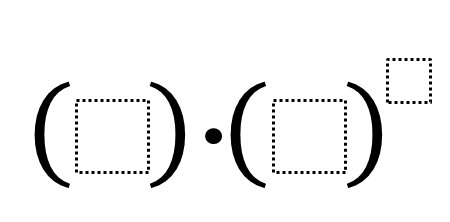# Exponents and Order of Operations

Directions: Find 3 positive integers that add up to 10. Place each number into one of the blanks to find the largest possible result.### Hint

Which part of the expression should be simplified first?
Which of the three boxes should get the largest number?

1 * 4^5

### Extensions

There are many extensions with the exact same prompt:
1. What’s the smallest possible result?
2. What if you’re working with all integers greater than (-5)?
3. How close can you get to 0 without hitting it exactly?

Source: Zack Miller (@zmill415)

## Square Root Expression 2

Directions: Using the digits 0 to 9 at most one time each, place a digit …

1.(2×3)^5 results in a larger number than (1×4)^5

•Notice that the exoponent only applies to the second base number.

2.Oops, didn’t see the comment thread above. The order of operations says we do the exponents first, so:
2 x 3^5 < 1 * 4^5

•I’m not sure if you noticed, Zack and Julie: Julie changed the position of the brackets. With that change, her answer is correct. However, with the original brackets, Zack is correct.

3.4.(2×3)^5 results in a larger number than (1×4)^5

5.6.(2×3)5<(1×40)*5

7.1 x 4^5

8.So on this one, are they allowed to repeat a digit? Is the question asking of the three numbers you chose what is the largest value you can get them to, or is it asking to find the best three digits that add up to 10 that would make the largest value?